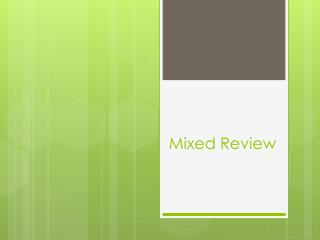DownloadDownload PresentationMixed Review

# Mixed Review

Télécharger la présentation## Mixed Review

- - - - - - - - - - - - - - - - - - - - - - - - - - - E N D - - - - - - - - - - - - - - - - - - - - - - - - - - -
##### Presentation Transcript

1. Mixed Review

2. Extra Credit Points: • AD Geometry - For every question correct on the DA you will receive one major grade extra credit point! • Pre-AP Geometry – For every question you get correct you will receive half a major grade extra credit point (rounded up). If you get all 17 correct you will get 9 points added to a major grade that will benefit you the most.

3. G.2B - 4 Questions • Make a conjecture about angles, lines, polygons, circle, and three dimentional figures and determine the validity of the conjectures, choosing from a variety of approaches such as coordinate, transformational, or axiomatic.

4. A Conjecture • A conclusion you reach by inductive reasoning (reasoning based on an observed pattern)

5. TEKS G.2B Question 1 . The figure below was formed by joining 2 segments of equal length at a common endpoint Y. . X Z . If the points X, Y, and Z are non-collinear, which of the following statements regarding XZ must always be true. Y A. XZ = XY B. XZ = 2(XY) C. XZ > 2(XY) D. XZ < 2(XY)

6. TEKS G.2B Question 2 . . W Y . Look at the diagram below. What conclusion can be drawnabout   YQX and WQZ Q . . Z X A. They are congruent B. They are adjacentC. They are supplementaryD. No conclusion can be drawn

7. TEKS G.2B Question 3 2 1 m 4 3 In the diagram, lines m and n are parallel. 5 6 n 8 7 A. 1 and 2 are complementaryB. 1 is congruent to 5 C. 2 and 8 are supplementaryD. 4 is congruent to 5

8. TEKS G.2B Question 4 Classify the triangle formed by connecting the following three points: ( 0, 7), ( 4, 7), ( 2, 3). Justify your answer using coordinate geometry. A. Scalene B. Isosceles C. Equilateral

9. G.3C - 4 Questions • Use logical reasoning to prove statements are true and find counter examples to disprove statements that are false.

10. TEKS G.3C Question 1 Which of these statements is a counter example for the statement “The area is always greater than the perimeter of a rectangle” A. The area of a rectangle with dimensions 2 units by 3 units is less than the perimeter of the same rectangle.B. The area of a rectangle with dimensions 4 units by 6 units is greater than the perimeter of the same rectangle.

11. TEKS G.3C Question 2 Which of the following is a counter example for the statement: “ If two lines are perpendicular to the same line, then they are always parallel to each other.” I. If two lines are perpendicular to the same line, they are skew to each other.II. If two lines are perpendicular to the same line, they are perpendicular to each other.A. I onlyB. II onlyC. Both I and II

12. TEKS G.3C Question 3 Reggie claimed that any number that is divisible by 3 is also divisible by 6. Which of the following examples disproves Reggie’s claim?A. 12B. 30C. 24D. 27

13. TEKS G.3C Question 4 Find a counter example for the conjecture “the square of any number is greater than the original number”A. -4B. 4C. -1D. 1

14. G.7B - 4 Questions • Use slopes and equations of lines to investigate geometric relationships, including parallel lines, perpendicular lines, and special segments of triangles and other polygons.

15. TEKS G.7B Question 1 RG is graphed on the coordinategrid to the right . Which of the following equations best represents the perpendicular bisect of RG ?

16. TEKS G.7B Question 2 Which equation represents a line parallel to AB?

17. TEKS G.7B Question 3 Which of the following best describes the graph of the equations below? A. Same x-interceptB. Same y-interceptC. The lines are parallel D. The lines are perpendicular

18. TEKS G.7B Question 4 A line contains the points ( 1, -3 ) and ( 2, 2 ). Which of the following pairs of points determine a line perpendicular? A. ( 0, 0 ) and ( -1, 5 )B. ( 0, 0 ) and ( 1, 5 )C. ( 1, 3 ) and ( 6, 2 )D. ( -4, 0 ) and ( 5, 5 )

19. G.7C - 5 Questions • Derive and use formulas involving length, slope, and midpoint.

20. TEKS G.7C Question 1 A coordinate grid is placed over a map. City A is located at ( -3, 2 ) and City B is located at ( 4, 8 ). If City C is at the midpoint between City A and City B, which is closest to the distance in coordinate units from City A to City C? A. 4.61 B. 6.52C. 9.22 D. 21.26

21. TEKS G.7C Question 2 S Which best represents the length of the shortest side of the triangle EHS? E H A. 5.5 B. 5.8C. 6.0 D. 6.3

22. TEKS G.7C Question 3 What is the length of the larger base on the trapezoid? A. 3.6 B. 10.8C. 4.5 D. 4

23. TEKS G.7C Question 4 A What is the midpoint of FB? B C E F A. ( 1, 1) B. ( -1, 1)C. ( 1, - 1) D. ( -1, -1) D

24. TEKS G.7C Question 5 A Which segments have the same slope? 1. BE 2. FE 3. BA 4. AF 5. CD B C E F D A. 3 and 2 only B. 1 and 5 onlyC. 4 and 5 and 3 only D. 5 and 1 and 4 only